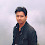### Matlab Code to Generate LoRa Symbols

In the previous post you read the theory about LoRa Symbol generation.

Code generates the following plot.

For theory please refer to  LoRa Symbol generation

If you are a research student and want to sell your work on my Blog here, please reach me on sakshama.ghosliya@gmail.com

1.Dear Sir,
In your LoRa modulation function code, the frequency varies linearly with time. Shouldn't the phase increase be a quadratic function of time, since the total phase is the integral of frequency? I'm very confused since your expression is not even a function of time. It would help me understand LoRa much better if you could clarify this point, Sir.

1.See, I divided the time in small time bins. You know, you can calculate the the symbol time by SF and BW. You can't see the 'time' variable in the code. See, you divided the complete symbol in some number of samples. Number of samples is related to SF and BW, therefore as the sample number increases you move along with both time and frequency axis.

2.I have multiple questions on this plot
1. Why is the plot at 500KHz when you only use BW, SF, Sampling rate in the code
2. what does frequency offset away from DC means?
3. How did you derive equation of number of samples from SF, BW, Fs?
4. Why is Fs = 1MSPS? is it lora standard?

1.Why is the plot at 500kHz? I see in the LoRa function: Frequency_Offset = (Fs/2) - (BW/2);

This calculation gives about 500kHz. But I think it should be the actual carrier frequency like 868MHz?

2.Why is the plot at 500kHz? I see in the LoRa function: Frequency_Offset = (Fs/2) - (BW/2);

This calculation gives about 500kHz. But I think it should be the actual carrier frequency like 868MHz?

3.If you want the central frequency to be 868MHz then you don't need to choose Frequency_Offset = (Fs/2) - (BW/2); In the above code it is 500KHz so that you can visualise the symbols clearly in the graph.

4.Can we directly put 868MHz instead of Frequency_Offset = (Fs/2) - (BW/2); in a code?

3.This comment has been removed by the author.

4.I would like to ask why you set the number of samples like the following num_samples = Fs*(2^SF)/BW? I know that the symbol time is Ts=(2^SF)/BW

5.i developed a matlab code to simulate lorawan throughput by using one gateway, any body can help to provide code of more than gateway in one network to make comparison

6.This comment has been removed by the author.

7.Dear Sir,
In your LoRa modulation function code, I think the " phase = phase + 2*pi*f/Fs;" should be changed to "phase = phase + 2*pi*f/Fs+pi*(BW*BW/Fs/Fs/2^SF);".

1.unless f0=0.5*BW^2/2^SF/Fs. Right?

8.Hi the variable symbol is any integer number our is it a bit been 1 or 0?

9.I am working on LoRa -modulation based communication . Kindly send me the matlab codes

10.What price og this? 10 Dollars or 15 dollars? On page "How to buy LoRa Matlab Codes?" - 15 dollars. On this page 10 dollars.

1.Hi. Sorry for the bug. I have fixed it now

11.hi what means by outp((i-1)*num_samples+1 : i*num_samples) = out_preamble;
outp what is?

1.outp is just a new variable in the code which concatenate the out_preamble data.